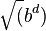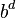# Alpha-beta pruning facts for kids

Kids Encyclopedia Facts

Alpha-beta pruning is a Search algorithm that tries to remove options that it can take. This algorithm is commonly used for a computer to play two player games, such as chess, go, and checkers. The algorithm stops analyzing a move when it finds another possible move that is proven to be better, and that move will not be analyzed again. This algorithm is a modification of minimax algorithm.

## Algorithm

The algorithm keeps track of two values, Alpha and Beta, and assigns "points" to possible moves where the greater the amount of points a move has, the higher chance that choosing that move will lead to victory. Alpha represents the minimum amount of points the player the algorithm wants to win can have(the maximizing player), while beta is the maximum amount of points the algorithm wants to lose can have(the minimizing player). Alpha and Beta start as negative infinity and infinity respectively. Whenever a move is found such that the maximizing player's score improves or the minimizing player's score decreases, then Alpha and Beta will be replaced with those new values.

In the best case, the complexity of the algorithm is O($\sqrt (b^d)$) while the worst case is O($b^d$) where b is the branching factor, that is how much each move splits into future options on average, and d is the depth, as in on average how many possible moves are made into the game.

## Images for kidsAlpha-beta pruning Facts for Kids. Kiddle Encyclopedia.